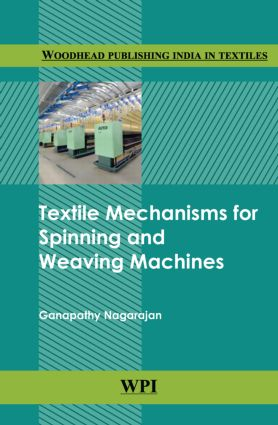# Textile Mechanisms in Spinning and Weaving Machines

## 1st Edition

WPI Publishing

384 pages | 225 B/W Illus.

##### Purchasing Options:\$ = USD
Hardback: 9789380308456
pub: 2014-12-14
\$250.00
x

FREE Standard Shipping!

### Description

The book has been written in response to numerous suggestions and great interest of colleagues and students in the textile industry to note the various design aspects in the mechanics of the textile machineries.

Preface

Foreword

Belt, rope and chain drives

Introduction

Selection of belt drive

Types of belt drives

Types of belts

Flat belt drives

Velocity ratio of belt drive

Power transmitted by a belt

Ratio of driving tensions for flat belt drive

Centrifugal tension

Initial tension in the belt

V-belt drive

Rope drive

Chain drives

Gear trains

Introduction

Nomenclature of gears

Rotary motion

Introduction

Linear displacement

Angular displacement

Speed

Transmission of rotary movement in machines

Classification of gears

Gears in textile machines

Equations of movement

Acceleration

Retardation

Parallelogram of velocities

Introduction

Velocity

Force, mass and momentum

Introduction

Definitions and units

Units of force

Momentum in quantity of motion

Work and power

Introduction

Units of work (in SI units)

Graphical representation of work

Useful definitions

Work done by a varying force

Resolution of forces

Introduction

Lines of representation of forces

Law of Polygon of forces

Law of Triangle of Forces

Tension and compression

Graphical methods

Resolution and composition of a force

Resolution of a number of coplanar forces

Centre of gravity

Introduction

Centre of gravity of irregular bodies

Centre of gravity of levers

Principle of moments

Moment of force

Geometrical representation of moments

Types of moments

Clockwise moments

Anti-clockwise moments

Varignon’s Principle of Moments or Law of Moments

Moments on bent lever

Moments of bent lever in weighting arrangement

Bent lever in weighting of single yarns

Bent lever on Lea strength tester

Friction

Introduction

Types of friction

Limiting friction

Coefficient of friction (Fig. 11.2)

Laws of solid friction

Friction of shaft in its bearings

Friction clutches

Power transmission

Coil friction

Introduction

Friction on curved surface

Coefficient of friction on solid surface

Transmission of power by belts and rope drives

Transmission of power by V-belts and rope drives

Brakes

Lifting machines and velocity ratio

Introduction

Definitions

Velocity ratio

Velocity ratio on simple pulleys

Weston differential pulley block

Types of machines

Law of a machine

Stress, strain and elasticity

Stress

Energy

Momentum energy impulsive forces and impact

Momentum

Law of Conservation of Momentum

Collision of two bodies

Collision of inelastic bodies

Collision of elastic bodies

Coefficient of Restitution (e)

Impulse

Energy

Potential energy

Kinetic energy

Strain energy

Law of Conservation of Energy

Motion in a circle

Introduction

Centripetal force

Centrifugal force

Conical pendulum

Tension in rotating masses

Simple harmonic motion

Introduction

Definitions of few terms

Simple pendulum

Helical springs

Moments of inertia and kinetic energy of rotating bodies

Mass moment of inertia

Fundamental equation for a rotating body

Kinetic energy of a rotating body

Compound pendulum

Centre of percussion

Practical methods of finding moment of inertia

Introduction

Flywheel

Methods of finding Moment of Inertia of fly wheels

Bifilar suspension

Referring Moment of Inertia

Moment of inertia referred to its driving shaft

Winding mechanics

Cams

Introduction

Classification of cams

Classification of followers

Cams in textile machines

Construction of the cam design

Terms in Cam profile

Scutcher feed regulating mechanism (Fig. 20.15)

Cone drum construction (Fig. 20.16)

Design of cone drum profile (Fig. 20.17)

Design of cone drums in speed frame (Fig. 20.18)

Tappets

Construction of shedding tappet

Determination of tappet lift

Determination of NPC and FPC

Relation between dwell period and type of heald movement

Tappet design for plain weave

Design of tappet for twill weave

Construction of a shedding tappet for three-shaft weave

Cranks and eccentrics

### Subject Categories

##### BISAC Subject Codes/Headings:
TEC021000
TECHNOLOGY & ENGINEERING / Material Science